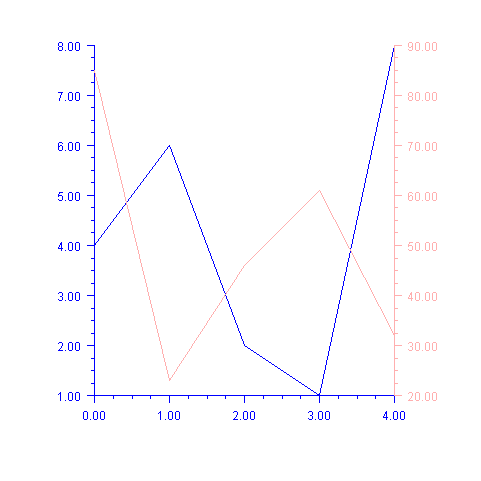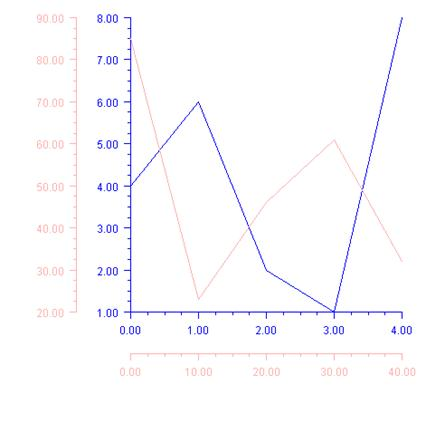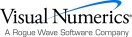## Multiple Axes

An IMSL C# Numerical Library chart can contain any number of axes. Each axis has its own mapping from the user coordinates to the device (screen) coordinates.

Normally, the x-axis is at the bottom of the chart and the y-axis is to the left. The attribute Type can be changed to move the x-axis to the top of the chart and/or the y-axis to the right of the chart.

Axis can be moved from the chart edge, either away from the chart or into the middle of the chart data area, by setting the attribute Cross.

#### Attribute Type

The attribute Type specifies the position of an x or y-axis.  Axis1D.Type should be used to set the axis type. Applied to the x-axis it can have the values AXIS_X (the default) or AXIS_X_TOP. Applied to the y-axis, it can have the value AXIS_Y (the default) or AXIS_Y_RIGHT.

#### Attribute Cross

The Cross attribute specifies the coordinates of the intersection of the x-axis and y-axis. AxisXY.SetCross should be used to set the coordinates. This can be inside or outside of the chart body. Cross can be used to place multiple y-axes to the left of the chart or multiple x-axes below the chart.

#### Example

Two data sets are plotted, each on its own axis. The first (blue) axis is left in the default position with the y-axis on the left. The second (pink) axis has its y-axis moved to the left.

In this example the x-axis is shared between the two axes, so it is not painted as part of the second (pink) axis. This is done by setting its IsVisible property attribute to false.using Imsl.Chart2D;

using System.Drawing;

public class SampleAxesLeftRight : FrameChart {

public SampleAxesLeftRight() {

Chart chart = this.Chart;

AxisXY axisLeft = new AxisXY(chart);

double[] yLeft = {4, 6, 2, 1, 8};

Data dataLeft = new Data(axisLeft, yLeft);

dataLeft.DataType = Data.DATA_TYPE_LINE;

axisLeft.LineColor = Color.Blue;

axisLeft.TextColor = Color.Blue;

AxisXY axisRight = new AxisXY(chart);

axisRight.AxisX.IsVisible = false;

axisRight.AxisY.Type = Axis1D.AXIS_Y_RIGHT;

double[] yRight = {85, 23, 46, 61, 32};

Data dataRight = new Data(axisRight, yRight);

dataRight.DataType = Data.DATA_TYPE_LINE;

axisRight.LineColor = Color.Pink;

axisRight.TextColor = Color.Pink;

}

public static void Main(string[] argv) {

System.Windows.Forms.Application.Run(

new SampleAxesLeftRight());

}

}

### Cross Example

Multiple x and y-axes are shown.

The Cross attribute is used to specify that the second (pink) axes intersect at  (-8,10), in the pink-axis coordinate system.

The Viewport attribute is changed in both sets of axes to shrink the size of the chart body and leave more room to the left and below the chart for the pink axes.using Imsl.Chart2D;

using System.Drawing;

public class SampleAxesCross : FrameChart {

public SampleAxesCross() {

Chart chart = this.Chart;

AxisXY axisLeft = new AxisXY(chart);

double[] yLeft = {4, 6, 2, 1, 8};

Data dataLeft = new Data(axisLeft, yLeft);

dataLeft.DataType = Data.DATA_TYPE_LINE;

axisLeft.LineColor = Color.Blue;

axisLeft.TextColor = Color.Blue;

AxisXY axisRight = new AxisXY(chart);

axisRight.SetCross(-8, 10);

double[] xRight = {0, 10, 20, 30, 40};

double[] yRight = {85, 23, 46, 61, 32};

Data dataRight = new Data(axisRight, xRight, yRight);

dataRight.DataType = Data.DATA_TYPE_LINE;

axisRight.LineColor = Color.Pink;

axisRight.TextColor = Color.Pink;

double[] viewport = {0.3, 0.9, 0.05, 0.7};

axisLeft.SetViewport(viewport);

axisRight.SetViewport(viewport);

}

public static void Main(string[] argv) {

System.Windows.Forms.Application.Run(new SampleAxesCross());

}

}Visual Numerics - Developers of IMSL and PV-WAVEhttp://www.vni.com/PHONE: 713.784.3131FAX:713.781.9260